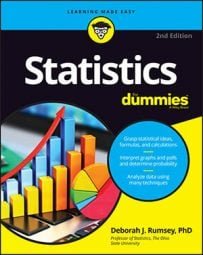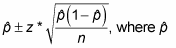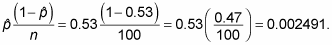##### Statistics For DummiesYou can find the confidence interval (CI) for a population proportion to show the statistical probability that a characteristic is likely to occur within the population.

When a characteristic being measured is categorical — for example, opinion on an issue (support, oppose, or are neutral), gender, political party, or type of behavior (do/don’t wear a seatbelt while driving) — most people want to estimate the proportion (or percentage) of people in the population that fall into a certain category of interest.

For example, consider the percentage of people in favor of a four-day work week, the percentage of Republicans who voted in the last election, or the proportion of drivers who don’t wear seat belts. In each of these cases, the object is to estimate a population proportion, p, using a sample proportion, ρ, plus or minus a margin of error. The result is called a confidence interval for the population proportion, p.

The formula for a CI for a population proportion isis the sample proportion, n is the sample size, and z* is the appropriate value from the standard normal distribution for your desired confidence level. The following table shows values of z* for certain confidence levels.

Confidence Level z*-value z*-values for Various Confidence Levels 80% 1.28 90% 1.645 (by convention) 95% 1.96 98% 2.33 99% 2.58
To calculate a CI for a population proportion:
1. Determine the confidence level and find the appropriate z*-value.

Refer to the above table for z*-values.

2. Find the sample proportion, ρ, by dividing the number of people in the sample having the characteristic of interest by the sample size (n).

Note: This result should be a decimal value between 0 and 1.

3. Multiply ρ(1 - ρ) and then divide that amount by n.

4. Take the square root of the result from Step 3.

This step gives you the margin of error.

6. Take ρ plus or minus the margin of error to obtain the CI; the lower end of the CI is ρ minus the margin of error, and the upper end of the CI is ρ plus the margin of error.

The formula shown in the above example for a CI for p is used under the condition that the sample size is large enough for the Central Limit Theorem to be applied and allow you to use a z*-value, which happens in cases when you are estimating proportions based on large scale surveys. For small sample sizes, confidence intervals for the proportion are typically beyond the scope of an intro statistics course.

For example, suppose you want to estimate the percentage of the time (with 95% confidence) you’re expected to get a red light at a certain intersection. Suppose you take a random sample of 100 different trips through this intersection and you find that a red light was hit 53 times.
1. Because you want a 95 percent confidence interval, your z*-value is 1.96.

2. The red light was hit 53 out of 100 times. So ρ = 53/100 = 0.53.

3. Find4. Take the square root to get 0.0499.

The margin of error is, therefore, plus or minus 1.96 ∗ 0.0499 = 0.0978, or 9.78%.

5. Your 95 percent confidence interval for the percentage of times you will ever hit a red light at that particular intersection is 0.53 (or 53 percent), plus or minus 0.0978 (rounded to 0.10 or 10%).

(The lower end of the interval is 0.53 – 0.10 = 0.43 or 43 percent; the upper end is 0.53 + 0.10 = 0.63 or 63 percent.)

To interpret these results within the context of the problem, you can say that with 95 percent confidence the percentage of the times you should expect to hit a red light at this intersection is somewhere between 43 percent and 63 percent, based on your sample. You might want to try a different route!